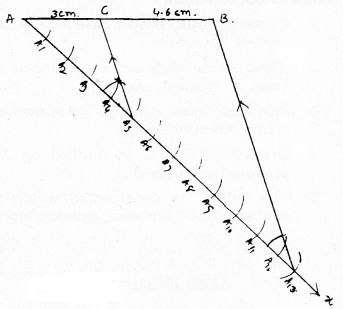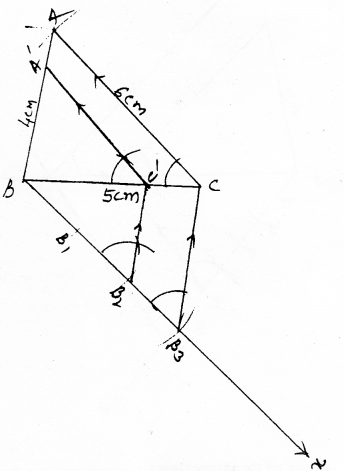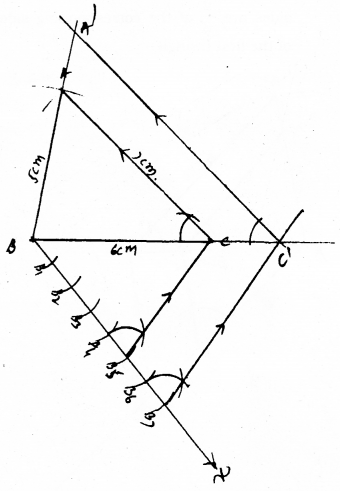# KSEEB SSLC Class 10 Maths Solutions Chapter 6 Constructions Ex 6.1

KSEEB SSLC Class 10 Maths Solutions Chapter 6 Constructions Ex 6.1 are part of KSEEB SSLC Class 10 Maths Solutions. Here we have given Karnataka SSLC Class 10 Maths Solutions Chapter 6 Constructions Exercise 6.1.

## Karnataka SSLC Class 10 Maths Solutions Chapter 6 Constructions Exercise 6.1

In each of the following, give the justification of the construction also :
Question 1.
Draw a line segment of length 7.6 cm and divide it in the ratio 5 : 8. Measure the two parts.
Solution:
Divide a line segment of 7.6 cm length in the ratio 5 : 8 and measure.
m : n = 5 : 8
m + n = 5 + 8 = 13
AC : CB = 5 : 8.
by measurement: AC = 3cm, CB = 4.6cm.Steps of Construction:

1. Draw any ray AB, such that AB = 7.6 cm.
2. Draw Ax ray at point A making acute angle.
3. Locate the points A1, A2, A3, ………., A13 from A.
4. Join BA13. Draw BA13 || A5C.
Now, AC : CB = 5 : 8
If measured, AC = 3 cm, CB = 4.6 cm.

Question 2.
Construct a triangle of sides 4 cm, 5 cm and 6 cm and then a triangle similar to it whose sides are $$\frac{2}{3}$$ of the corresponding sides of the first triangle.
Solution:
Construct a triangle ABC having sides 4 cm, 5 cm and 6 cm. For this we have to construct a triangle similar to it whose sides are $$\frac{2}{3}$$ of the corresponding sides of the first triangle.Steps of Construction:

1. ∆ABC is constructed, having AB = 4 cm, BC = 5 cm, CA = 6 cm.
2. Draw Bx ray such that it forms acute angle to BC which is adjacent to vertex A.
3. Locate 3 points on Bx such that BB1 = B1B2 = B2B3.
4. Join B3C. Draw a parallel line to B3C which intersect BC at C.
5. Draw a parallel line through C’ to CA and it intersects BA at A’.
Now, A’BC’ is required triangle.

Question 3.
Construct a triangle with sides 5 cm, 6 cm and 7 cm and then another triangle whose sides are $$\frac{7}{5}$$ of the corresponding sides
of the first triangle.
Solution:
Construct an ∆ABC having sides 5 cm, 6 cm and 7 cm. Then construct another triangle whose sides are $$\frac{7}{5}$$ of the corresponding sides of the first triangle.Steps of Construction :

1. First construct a triangle ABC having sides AB = 5cm, BC = 6 cm, CA = 7 cm.
2. Draw a ray Bx such that it makes an acute angle at ‘B’.
3. Locate Points B1, B2, B3, B4, B5, B6, By points on Bx.
4. Join B5C. Draw B5C || B7C’, it intersects at ‘C’ which is produced BC line.
5. Draw AC || C’A’, it meets produced line BC at A’.
6. Now required ∆A’BC’ is obtained whose sides are $$\frac{7}{5}$$ of the corresponding sides of the first triangle.

Question 4.
Construct an isosceles triangle whose base is 8 cm and altitude 4 cm and then another triangle whose sides are $$1 \frac{1}{2}$$ times the corresponding sides of the isosceles triangle.
Solution:
Construct an isosceles triangle with base 8 cm and altitude 4 cm and then another triangle whose sides are $$1 \frac{1}{2}$$ times the corresponding sides of the isosceles triangle.Steps of Construction:

1. Construct ∆ABC having base AB = 8 cm, altitude AD = 4 cm.
2. ∠BAx acute angle is constructed at A. From ‘A’ locate A1, A2, A3.
3. Join A2B. Draw A2B || A3B’. B is marked on line AB produced.
4. Draw AB || B’C’. C’ is marked on AC produced and draw AC’.
5. Now ∆AB’C’ is constructed which is similar to ∆ABC and corresponding sides,
i.e., $$1 \frac{1}{2}=\frac{3}{2}$$
∆ABC ||| ∆AB’C’

Question 5.
Draw a triangle ABC with side BC = 6 cm, AB = 5 cm, and ∠ABC = 60°. Then construct a triangle whose sides are $$\frac{3}{4}$$ of the corresponding sides of the triangle ABC.
Solution:
Construct a triangle ABC with sides BC = 6 cm, AB = 5 cm and ∠ABC = 60°. Then construct a triangle whose sides are $$\frac{3}{4}$$ of the corresponding sides of the triangle ABC.Steps of Construction:

1. Construct a AABC having BC = 6 cm, AB = 5 cm, and ∠ABC = 60°.
2. Draw a ray Bx to form acute angle at B and mark BB1, B2, B3, at equal intervals of distance.
3. Join B4C. Draw B4C || B3C’.
4. Draw AC || A’C’.
5. Now ∆ABC’ is equal to ∆ABC.
∴ ∆A’BC’ ||| ∆ABC
3 : 4

Question 6.
Draw a triangle ABC with side BC = 7 cm, ∠B = 45°, ∠A = 105°. Then, construct a triangle whose sides are $$\frac{4}{3}$$ times the corresponding sides of ∆ABC
Solution:
Draw a triangle ABC with side BC = cm, ∠B = 45°, ∠A = 105°. The construct a triangle whose sides are $$\frac{4}{3}$$ times the corresponding sides of ∆ABC.Steps of Construction :

1. Construct a triangle ABC having BC = 7 cm, ∠A = 45° and ∠B = 105°.
2. Draw Bx ray at B to form acute angle.
3. Mark points B1, B2, B3, B4 from B. Draw B4C’.
4. Draw B4C || B3C’. Draw AC || A’C’.
5. Now, ∆A’BC’ ||| ∆ABC is constructed.
∆A’BC’ : ∆ABC

Question 7.
Draw a right triangle in which the sides (other than hypotenuse) are of lengths 4 cm and 3 cm. Then construct another triangle whose sides are $$\frac{5}{3}$$ times the corresponding sides of the given triangle.
Solution:
Draw a right triangle in which the sides (other than hypotenuse) are of lengths 4 cm and 3 cm. Then construct another triangle whose sides are $$\frac{5}{3}$$ times the corresponding sides of the given triangle.Steps of Construction:

1. Construct Right angled triangle ABC having sides BC = 4 cm, BA = 3 cm, and ∠B = 90°.
2. Draw a ray Bx to form acute angle at B.
3. Mark points B1, B2, B3, B4 from B at equal intervals.
4. Join B4C. Draw B4C || B3C’.
5. Draw AC ‘|| C’A’, then ∆A’BC’ is constructed.
6. Now, ∆A’BC’ ||| ∆ABC.
∴ ∆A’BC’ : ∆ABC = 5 : 3.

We hope the given KSEEB SSLC Class 10 Maths Solutions Chapter 6 Constructions Ex 6.1 will help you. If you have any query regarding Karnataka SSLC Class 10 Maths Solutions Chapter 6 Constructions Exercise 6.1, drop a comment below and we will get back to you at the earliest.

error: Content is protected !!